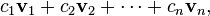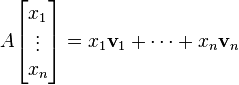# Column space

Let A be an m × n matrix, with column vectors v1v2, …, vn. A linear combination of these vectors is any vector of the formwhere c1c2, …, cn are scalars. The set of all possible linear combinations of v1,…,vn is called the column space of A. That is, the column space of A is the span of the vectors v1,…,vn.

Example
If, then the column vectors are v1 = (1, 0, 2)T and v2 = (0, 1, 0)T.
A linear combination of v1 and v2is any vector of the formThe set of all such vectors is the column space of A. In this case, the column space is precisely the set of vectors (xyz) ∈ R3 satisfying the equation z = 2x (using Cartesian coordinates, this set is a plane through the origin in three-dimensional space).

Any linear combination of the column vectors of a matrix A can be written as the product of A with a column vector:Therefore, the column space of A consists of all possible products Ax, for x ∈ Rn. This is the same as the image (or range) of the corresponding matrix transformation.